# Minimum Values: Definition & Concept

Lesson Transcript
Instructor
Jennifer Beddoe

Jennifer has an MS in Chemistry and a BS in Biological Sciences.

Expert Contributor
Kathryn Boddie

Kathryn has taught high school or university mathematics for over 10 years. She has a Ph.D. in Applied Mathematics from the University of Wisconsin-Milwaukee, an M.S. in Mathematics from Florida State University, and a B.S. in Mathematics from the University of Wisconsin-Madison.

The minimum value of a quadratic function is the low point at which the function graph has its vertex. This lesson will define minimum values and give some example problems for finding those values. A quiz will complete the lesson. Updated: 08/05/2020

## Exercise

The minimum value of a function is the place where the graph has a vertex at its lowest point. In the real world, you can use the minimum value of a quadratic function to determine minimum cost or area. It has practical uses in science, architecture and business.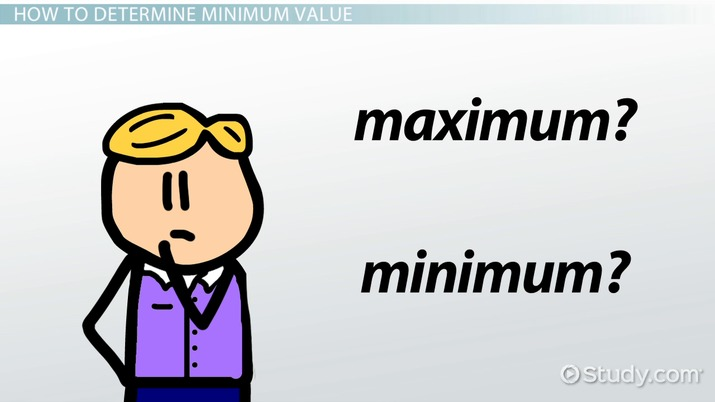An error occurred trying to load this video.

Try refreshing the page, or contact customer support.

Coming up next: Mode: Definition & Sample Problems

### You're on a roll. Keep up the good work!

Replay
Your next lesson will play in 10 seconds
• 0:00 Exercise
• 0:18 How to Determine Minimum Value
• 2:36 Real World Examples
• 4:01 Lesson Summary
Save Save

Want to watch this again later?

Timeline
Autoplay
Autoplay
Speed Speed

## How to Determine Minimum Value

There are three methods for determining the minimum value of a quadratic equation. Each of them can be useful in determining the minimum.

The first way is by using a graph. You can find the minimum value visually by graphing the equation and finding the minimum point on the graph. The y-value of the vertex of the graph will be the minimum. This is especially easy when you have a graphing calculator that can do most of the work for you.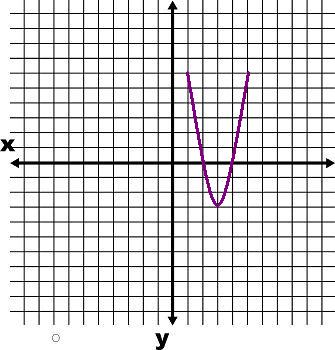Looking at this graph, you can see that the minimum point of the graph is at y = -3.

The second way to find the minimum value comes when you have the equation y = ax^2 + bx + c. If your equation is in the form y = ax^2 + bx + c, you can find the minimum by using the equation min = c - b^2/4a.

The first step is to determine whether your equation gives a maximum or minimum. This can be done by looking at the x^2 term. If this term is positive, the vertex point will be a minimum; if it is negative, the vertex will be a maximum. After determining that you actually will have a minimum point, use the equation to find it.

Let's do an example. Find the minimum point of 3x^2 + 12x + 2.

Since the term with the x^2, or 'a' term, is positive, you know there will be a minimum point. To find it, plug the values into the equation min = c - b^2/4a.

That gives us min = 2 - 12^2/4(3)

This simplifies to min = 2 - 144 / 12, which can be further simplified to min = 2 - (12), or min = -10.

The third way to find the minimum value is using the equation y = a(x - h)^2 + k.

As with the last equation, the a term in this equation must be positive for there to be a minimum. If the a term is positive, the minimum can be found at k. No equation or calculation is necessary; the answer is just k.

Let's look at an example and find the minimum of the equation (x + 13)^2 + 2.

Since the a term is positive, there will be a minimum at y = 2.

To unlock this lesson you must be a Study.com Member.

## Practice Problems - Finding Minimum Values

In the following practice problems, students will find the minimum value of a quadratic function by using a graph, using the formula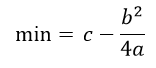and by just reading the value k when the function is written in the form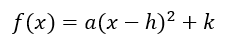## Practice Problems

1. Find the minimum value of the function using all three methods: looking at the graph, using the formulaand reading the value k from the formDo all the answers agree? Should they agree?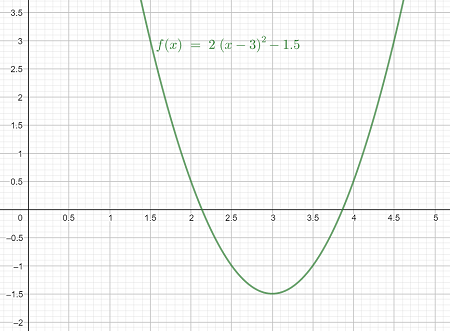2. A business is wanting to minimize their manufacturing costs. It costs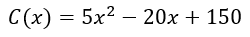in thousands of dollars to produce x thousand units of the commodity. What is the minimum cost for production?

3. Find the minimum value of the function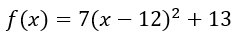## Solutions

1. The minimum value is the y value of the lowest point on the graph. Looking at the graph, this is -1.5.

The function is given in the formwhere k = -1.5, so using this method agrees with the graph method.

To use the formulawe need to first distribute to find the values of a, b, and c. We have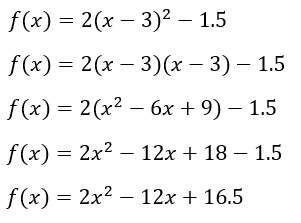So a = 2 , b = -12, c = 16.5. Using the formula, we find that the minimum is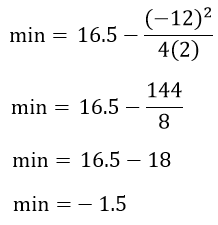which also agrees with the other methods. The answers should agree, since they are all methods to find the same value - which method is easier to use depends on the situation.

2. Since the cost function is written in the proper form to use the formulawe will find the minimum cost that way. We have a = 5, b = -20, and c = 150 and so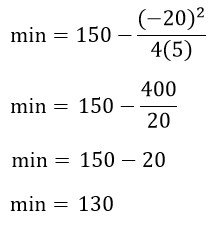The minimum cost is 130 thousand dollars.

3. This function is written in the formand so the minimum value is k = 13.

### Register to view this lesson

Are you a student or a teacher?